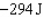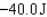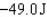/
/
/
16. A 1 200-kg automobile moving at 25 m/s has
Not my Question
Flag Content

# Question :   16. A 1 200-kg automobile moving at 25 m/s has : 2102118

16. A 1 200-kg automobile moving at 25 m/s has the brakes applied with a deceleration of 8.0 m/s2. How far does the car travel before it stops?

A. 39 m

B. 47 m

C. 55 m

D. 63 m

17. If during a given physical process the only force acting on an object is friction, which of the following must be assumed in regard to the object's kinetic energy?

A. It decreases.

B. It increases.

C. It remains constant.

D. It cannot be determined from the information given.

18. A 50.0-kg (including the passenger) sled is subject to a net force of 20.0 N pushing in the direction of the sled’s motion as it is moving over a horizontal surface for a distance of 11.0 m after having started from rest. At this point the sled is released as it starts down a 10.0? incline. However, the snow is not very deep, and the sled stops after having moved an additional 35.0 m. What is the work done by friction while the sled is on the incline?

A. –220 J

B. –3200 J

C. –858 J

D. –2980 J

19. A 10.0-kg sled slides down a snowy hill. At position A it is moving at 1.00 m/s. when it reaches position B it is moving at 3.00 m/s. Finally it passes position C moving at 1.00 m/s again. Position C is 3.00 m lower than position A and 1.00 m lower than position B.The coefficient of kinetic friction varies from place to place in the snow. What is the work done by friction on the sled as it moves from A to C?

A.B.C.D. Insufficient information is given to solve this problem.

20. A very light cart holding a 300-N box is moved at constant velocity across a 15-m level surface. What is the net work done in the process?

A. zero

B. 1/20 J

C. 20 J

D. 2 000 J

## Solution 5 (1 Ratings )

Solved
Physics 8 Months Ago 120 Views